# Force

Pushing or pulling any body in a given direction is called as applying force to the body.

Just like Messi kicks the ball in different directions.

# Effects of Force.

Force can change the speed of an object.

If the speed of an object is 0, it can be given some speed using force.

An object in motion can be stopped using a force.

A force can also change the direction of a moving object.

Sometimes, objects change shape when a force is applied on them.

That is another effect of application of a force.

# Directional Forces.

When two or more people push a car, each of them apply individual forces on the car, in the same direction.

All these forces add up, since they are in the same direction.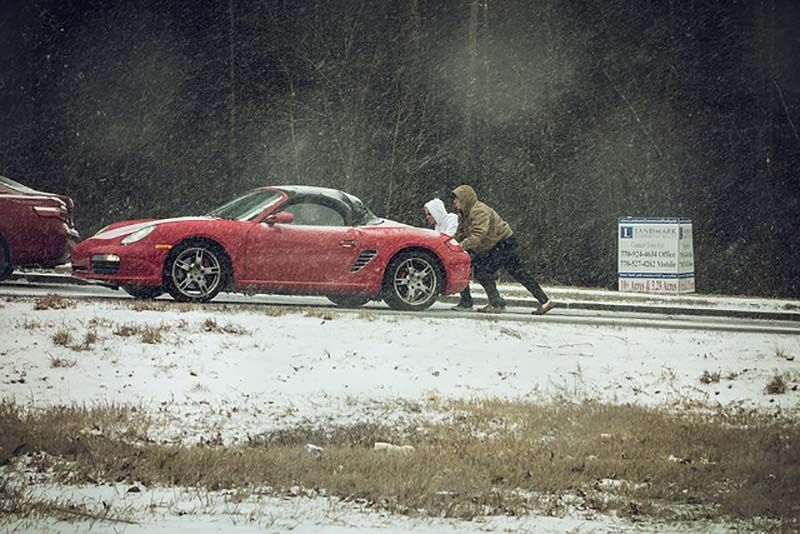But when two forces are in opposite direction, the forces get subtracted.

For instance, in this game of 'Tug of War', both teams pull the same rope in opposite directions.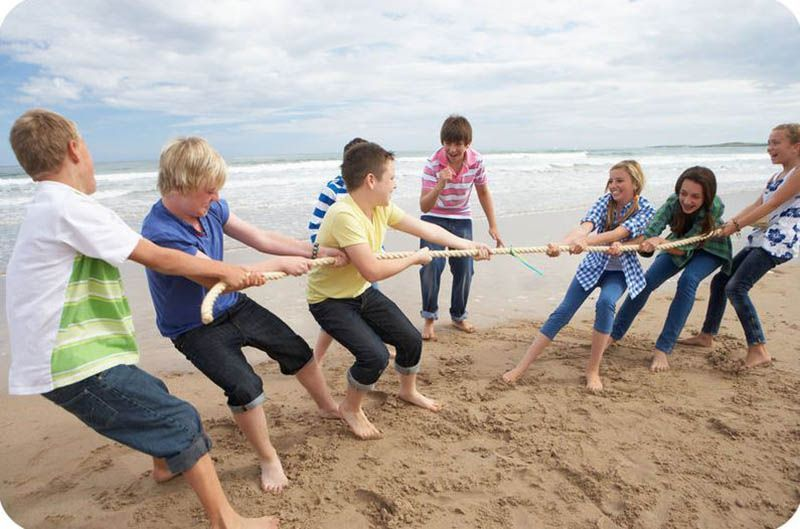Till both the teams apply the same force, the forces subtract from each other to become 0. The rope doesn't move in that case.

When one team applies more force in their direction, they pull the other team by extra force. That is when they are declared the winner.

So when two opposite forces are applied on a body, the body moves in the direction of the greater force.

# A Newton.

Force is measured in a unit called Newton (N).

It is the force that increases the speed of a 1kg object, by 1 m/s, every second.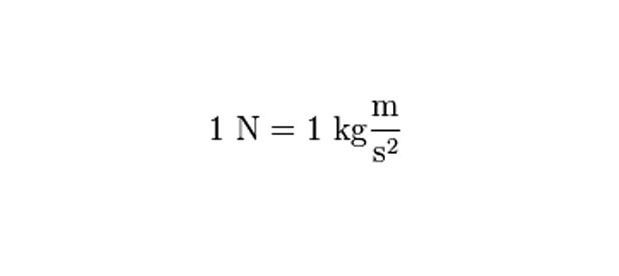Fact:
Earth pulls a 1kg mass with a force of 9.8N.

Just how mass is measured in 'kilograms (kg)', volume is measured in 'litres (l)', force is measured in 'Newton (N)'.

Let us look at a sample problem now.

# Access All Content

## More from Grade 5 Science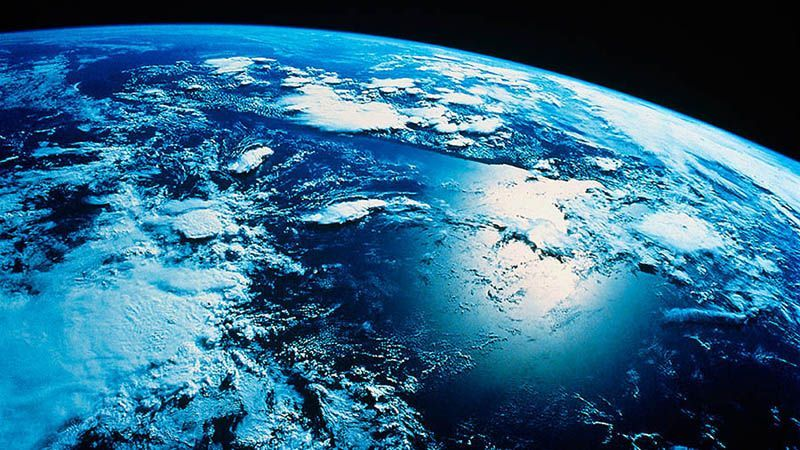# Water

Understand the concepts of water on earth, its sources and groundwater.# Photosynthesis

How do plants make their food? Get inside the plant to understand the process of photosynthesis.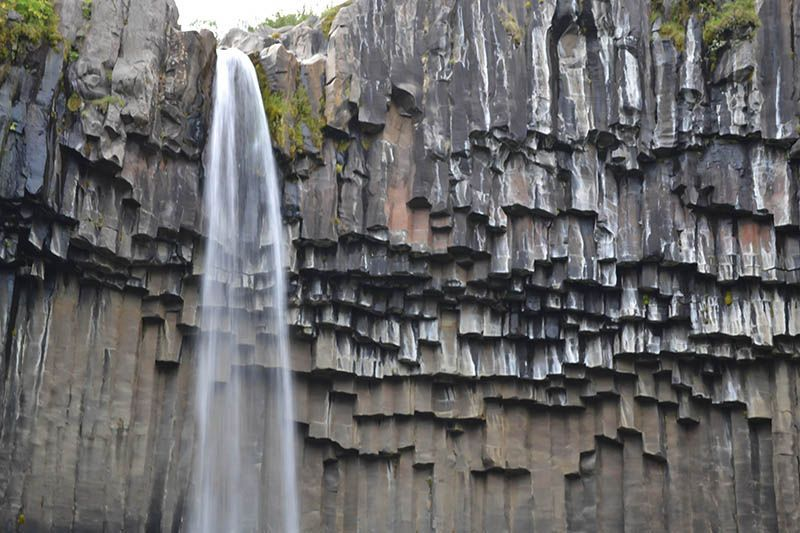# Rocks

Visit the depths of the Earth to see the birth of a rock! Learn about the different types of rocks, igneous, sedimentary and metamorphic, and how they're formed.